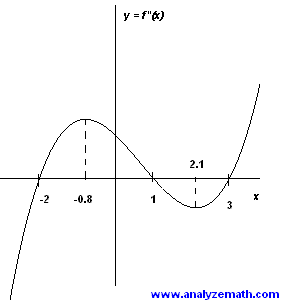# Calculus Questions with Answers (2)

Calculus questions with detailed solutions are presented. The behaviors and properties of functions, first derivatives and second derivatives are studied graphically.

### Question 1

The graphs of function f, its first f ' and second derivatives f", are shown below. Identify the graph of function f, the graph of its first derivative f ' and the graph of its second derivative f".Solution to Question 1:

• Graph (I) is above the x axis and therefore cannot be the derivative of either (II) or (III) since these have intervals of increase and decrease and their derivatives must change signs. So graph (I) is the graph of function f.
• Graph (I) has a maximum at x = 0 and its first derivative must be zero at x = 0. Hence only graph (III) satisfies this condition. Graph (III) is that of f '. Finally graph (II) can only be f " since f " is negative for values of x for which f ' is decreasing and positive for values of x for which f ' is increasing. Also f" is negative around x = 0 which corresponds to the fact that the graph of f is concave down around x = 0.

### Question 2

The graph of the first derivative f ' of function f is shown belowa) For what values of x is f increasing?
b) For what values of x is f decreasing?
c) For what value(s) of x does f have a local maximum or minumum?
d) For what value(s) of x is the graph of f concave up? concave down?
e) Where are the points of inflection of the graph of f located?

Solution to Question 2:

• a) f is increasing for values of x for which f ' is positive. The set of these x values is given by the intervals
(-infinity , -6.6) , (0 , 3.6)
• b) f is decreasing for values of x for which f ' is negative. The set of these x values is given by the intervals
(-6.6 , 0) , (3.6 , +infinity)
• c) f has a local maximum at values of x for which f '(x) = 0 and f ' changes from positive to negative. Hence f has a maximum at
x = -6.6 and x = 3.6
• f has a local minimum at values of x for which f '(x) = 0 and f ' changes from negative to positive. Hence f has a maximum at
x = 0
• d) The concavity is determined by the sign of f". Using the graph of f ' shown above, we can deduce the following:
1 - for the values of x in the interval (-4 , 2) f ' is increasing and therefore f" is positive. Hence f is concave up for values of on (-4 , 2)
2 - for the values of x in the intervals (-infinity , -4) and (2 , +infinity) f ' is decreasing and therefore f" is negative. Hence f is concave down for values of on (-infinity , -4) U (2 , +infinity).
• e) Again using the graph of f ', f"(x) = 0 for x = -4 and x = 2 since f ' has extrema at these two values of x. These are also the values for which f" changes and are therefore points of inflection.

### Question 3

The graph of the second derivative f " of function f is shown belowa) For what value(s) of x does f ' have a local maximum or minimum?
b) For what value(s) of x is the graph of f concave up?
c) For what value(s) of x is the graph of f concave down?
d) Where are the points of inflection of the graph of f located?

Solution to Question 3:

• a) f '(x) has a local maximum for values of x such that f"(0) and f" changes from positive to negative. There is only one such a point: x = 1. f '(x) has a local minimum for values of x such that f"(0) and f" changes from negative to positive. There are two values: x = -2 and x = 3
• b) f is concave up for values of x such that f" is positive. According to given graph f" is positive on interval (-2 , 1) and (3 , +infinity).
• c) f is concave down for values of x such that f" is negative. Using above graph, f" is negative on (-infinity , -2) and (1 , 3).
• d) Points of inflection are located at values of x where f" changes sign. Using the graph, the points of inflection are located at x = -2, 1 and 3.

| 1 | 2 | 3 | 4 | 5 |

More on calculus
questions with answers, tutorials and problems .